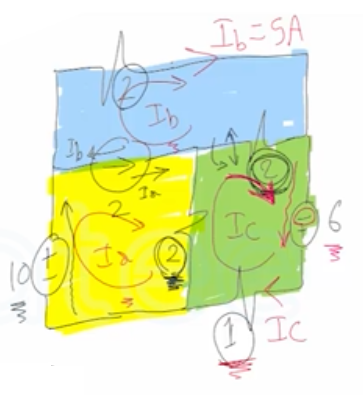Need Help?

Subscribe to Circuit

###### \${selected_topic_name}
• Notes
• Comments & Questions

$\begin{array}{l}{\text { Use the mesh-current method to find the }} \\ {\text { power dissipated in the } 1 \Omega \text { resistor in the cir- }} \\ {\text { cuit shown. }}\end{array}$$\text { super mesh }$

$2=I_{a}-I_{b} \longrightarrow (1)$

$2I_a-2I_c+4I_b-2I_c-10=0\longrightarrow (2)$

$\text { mesh (c) }$

$(1+2+2) I_{c}-2I_a-2I_b-6=0 \longrightarrow (3)$

$I_a=7A \quad , I_b=5A \quad, I_c=6A$
$\text { direction all correct }$

$P_{1 \Omega}=I^2 R=I_{c}^{2}*1=(6)^2*1=36w$
$\text { dissipated }$

No comments yet